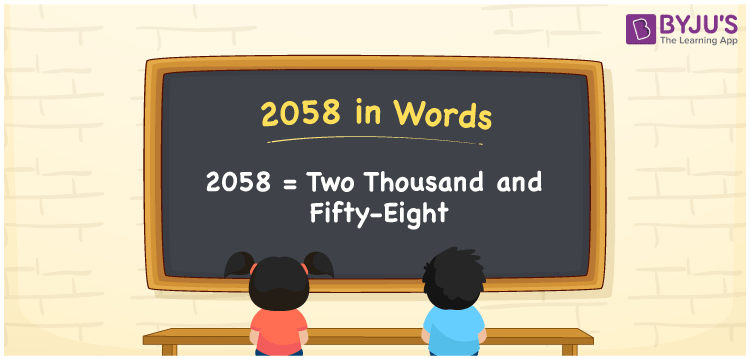# 2058 in Words

2058 in words is written as Two thousand fifty-eight. In both the International System of Numerals and the Indian System of Numerals, 2058 is written as Two thousand fifty-eight. The number 2058 is a Cardinal Number as it could represent some quantity.

 2058 in Words Two thousand fifty-eight Two thousand fifty-eight in Number 2058

## 2058 in English Words

2058 in English words is read as “Two thousand fifty-eight”.## How to Write 2058 in Words?

To write 2058 in words, we shall use the place value chart. In the place value chart, put 2 in the thousands, 0 in the hundreds, 5 in the tens, and 8 in the ones, respectively. Let us make a place value chart to write the number 2058 in words.

 Thousands Hundreds Tens Ones 2 0 5 8

Thus, we can write the expanded form as

2 × Thousand + 0 × Hundred + 5 × Ten + 8 × One

= 2 × 1000 + 0 × 100 + 5 × 10 + 8 × 1

= 2000 + 0 + 50 + 8

= 2058

= Two thousand fifty-eight.

2058 is a natural number, the successor of 2057 and the predecessor of 2059.

2058 in words – Two thousand fifty-eight

• Is 2058 an odd number? – No
• Is 2058 an even number? – Yes
• Is 2058 a perfect square number? – No
• Is 2058 a perfect cube number? – No
• Is 2058 a prime number? – No
• Is 2058 a composite number? – Yes

## Frequently Asked Questions on 2058 in Words

Q1

### How to write 2058 in words?

2058 in words is written as Two thousand fifty-eight.
Q2

### How to write 2058 in the International and Indian System of Numerals?

In both, the system of numerals, 2058 in words, is written as Two thousand fifty-eight.
Q3

### How to write 2058 in a place value chart?

In the place value chart, write 2 in the thousands, 0 in the hundreds, 5 in the tens, and 8 in the ones, respectively.